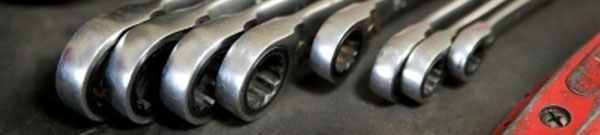# Fundamentals of Automotive electricityElectrical Circuit

Electrical devices are used in numerous areas of an automobile, and provide various functions.
As electricity passes through a resistor, it affects the resistor and can provide a number of functions.
Electrical devices utilize those functions according to purpose by converting electricity into work.
Functions of electricity
1. Heat-generation function
Heat is generated as electricity passes through a resistor, such as a cigarette lighter, fuse.
2. Light-emitting function
Light is emitted as electricity passes through a resistor, such as a light bulb.
3. Magnetic function
A magnetic force is generated as electricity passes through a conductor or coil, such as an ignition coil, alternator, injector.

All substances are comprised of atoms, which consist of nuclei and electrons. A metallic atom contains free electrons.
Free electrons are electrons that can move freely from the atoms.
The transfer of these free electrons among metallic atoms generates electricity.
Therefore, the electricity flow through an electrical circuit is the electrons moving in a conductor.
When a voltage is applied to both ends of a metal (conductor), the electrons flow from the negative pole to the positive pole. This flow is the opposite of the flow of an electrical current.
Three elements of electricity
Electricity consists of three basic elements:
1. Current
This is the current flow through an electrical circuit.
Unit: A (ampere)
2. Voltage
This is the force of electricity that moves current through an electrical circuit. The higher the voltage, the greater the amount of current that will flow through the circuit.
Unit: V (volt)
3. Resistance
This is the opposition to the current flow.
Unit: Ω (ohm)